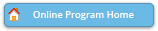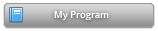#### Abstract Details

 Activity Number: 499 - Section on Nonparametric Statistics - Student Paper Competition Presentations Type: Topic Contributed Date/Time: Wednesday, July 31, 2019 : 10:30 AM to 12:20 PM Sponsor: Section on Nonparametric Statistics Abstract #301839 Presentation Title: More Efficient Computation of Smoothing Splines via Space-Filling Basis Selection Author(s): Cheng Meng* Companies: Keywords: Space-filling design; Star discrepancy; Nonparametric regression; Penalized least squares; basis selection Abstract: Smoothing spline is a flexible approach for nonparametric regression. For a sample of size n, the smoothing spline estimator is a linear combination of n basis functions, requiring O(n^3) computational time when the number of predictors d>1. Such sizable computational cost hinders smoothing splines of the broad applications. In practice, the full sample smoothing spline estimator can be approximated by the estimator based on q randomly-selected basis functions, leading to a computational cost of O(nq^2). It is known that these two estimators converge at the same rate when q is roughly at the order of O(n^{2/(pr+1)}), where p indicates on the smoothness of the true function, and r>0 relies on the type of the spline. Such q is called the essential number of basis functions. We develop a more efficient basis selection method. The proposed method chooses a set of basis functions with large ``diversity", by selecting the ones corresponding to roughly equal-spaced observations. Both the asymptotic analysis and the experimental studies show our proposed smoothing spline estimator reduce the essential number of basis functions q to roughly O(n^{1/(pr+1)}), when d< pr+1.

Authors who are presenting talks have a * after their name.